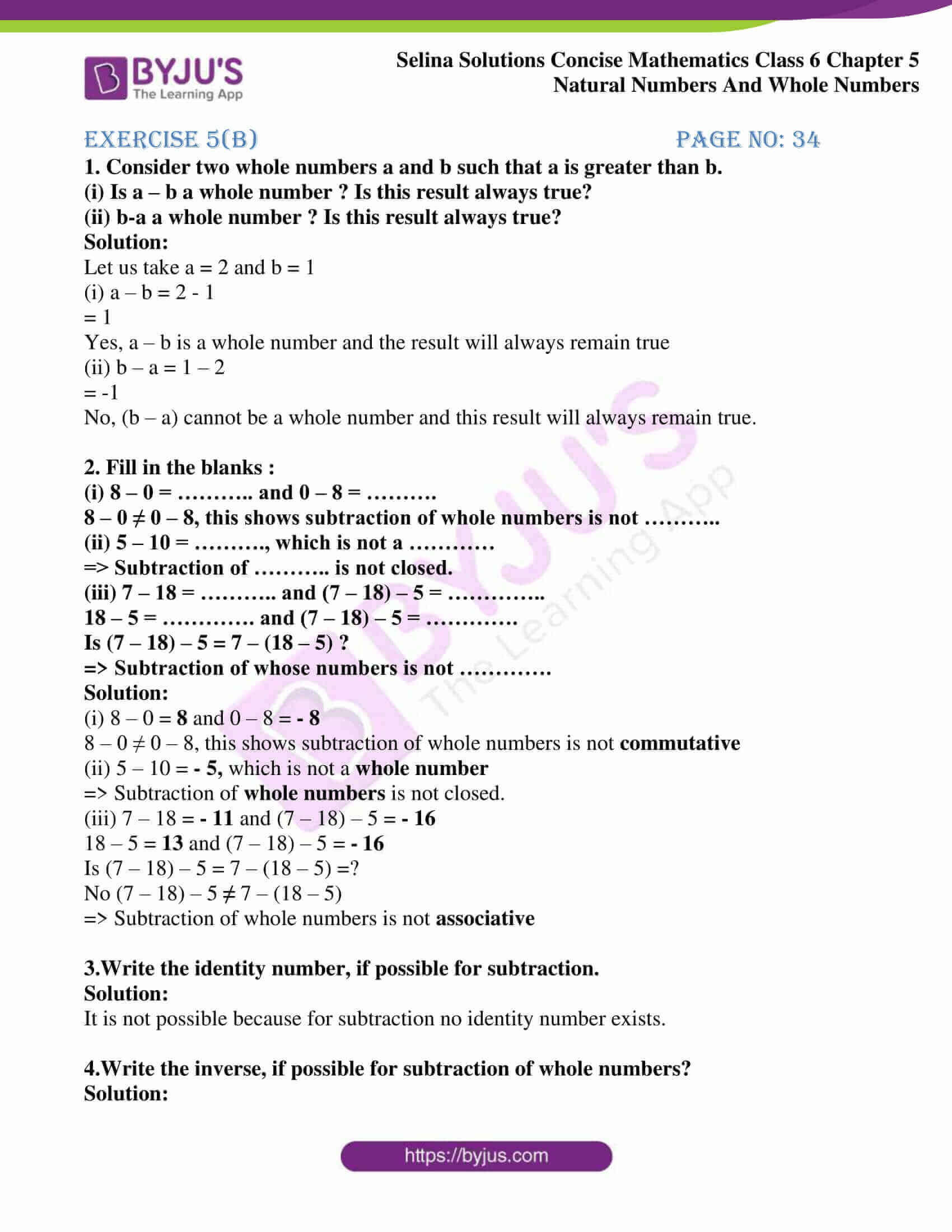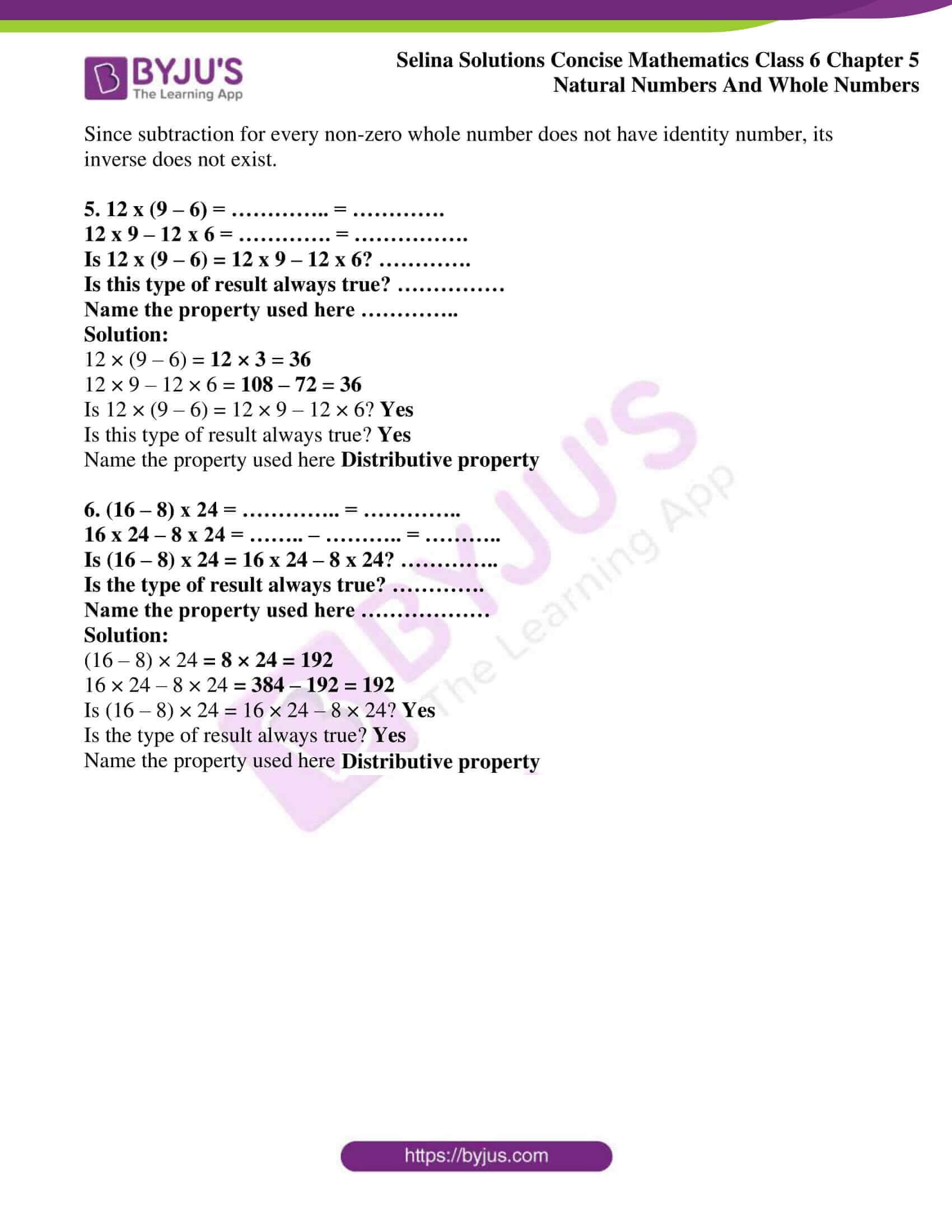# Selina Solutions Concise Mathematics Class 6 Chapter 5: Natural And Whole Numbers Exercise 5(B)

Selina Solutions Concise Mathematics Class 6 Chapter 5 Natural Numbers And Whole Numbers Exercise 5(B) explains the properties of Whole Numbers, as per the students’ understanding capacity. Practicing textbook questions on a daily basis helps students to gain a better hold on the concepts covered. Students who want to secure high marks in annual exams, need a thorough practice of Selina Solutions. The Solutions are prepared by the expert teachers at BYJU’S to help students in exam preparation. Access Selina Solutions Concise Mathematics Class 6 Chapter 5 Natural Numbers And Whole Numbers Exercise 5(B) PDF, from the links which are provided here

## Selina Solutions Concise Mathematics Class 6 Chapter 5: Natural Numbers And Whole Numbers Exercise 5(B) Download PDF### Access other exercises of Selina Solutions Concise Mathematics Class 6 Chapter 5: Natural Numbers And Whole Numbers

Exercise 5(A) Solutions

Exercise 5(C) Solutions

Exercise 5(D) Solutions

Exercise 5(E) Solutions

Exercise 5(F) Solutions

### Access Selina Solutions Concise Mathematics Class 6 Chapter 5: Natural Numbers And Whole Numbers Exercise 5(B)

#### Exercise 5(B) page no: 34

1. Consider two whole numbers a and b such that a is greater than b.
(i) Is a – b a whole number ? Is this result always true?
(ii) b-a a whole number ? Is this result always true?

Solution:

Let us take a = 2 and b = 1

(i) a – b = 2 – 1

= 1

Yes, a – b is a whole number and the result will always remain true

(ii) b – a = 1 – 2

= -1

No, (b – a) cannot be a whole number and this result will always remain true.

2. Fill in the blanks :
(i) 8 – 0 = ……….. and 0 – 8 = ……….
8 – 0 ≠ 0 – 8, this shows subtraction of whole numbers is not ………..
(ii) 5 – 10 = ………., which is not a …………
=> Subtraction of ……….. is not closed.
(iii) 7 – 18 = ……….. and (7 – 18) – 5 = …………..
18 – 5 = …………. and (7 – 18) – 5 = ………….
Is (7 – 18) – 5 = 7 – (18 – 5) ?
=> Subtraction of whose numbers is not ………….

Solution:

(i) 8 – 0 = 8 and 0 – 8 = – 8

8 – 0 ≠ 0 – 8, this shows subtraction of whole numbers is not commutative

(ii) 5 – 10 = – 5, which is not a whole number

=> Subtraction of whole numbers is not closed.

(iii) 7 – 18 = – 11 and (7 – 18) – 5 = – 16

18 – 5 = 13 and (7 – 18) – 5 = – 16

Is (7 – 18) – 5 = 7 – (18 – 5) =?

No (7 – 18) – 5 7 – (18 – 5)

=> Subtraction of whole numbers is not associative

3.Write the identity number, if possible for subtraction.

Solution:

It is not possible because for subtraction no identity number exists.

4.Write the inverse, if possible for subtraction of whole numbers?

Solution:

Since subtraction for every non-zero whole number does not have identity number, its inverse does not exist.

5. 12 x (9 – 6) = ………….. = ………….
12 x 9 – 12 x 6 = …………. = …………….
Is 12 x (9 – 6) = 12 x 9 – 12 x 6? ………….
Is this type of result always true? ……………
Name the property used here …………..

Solution:

12 × (9 – 6) = 12 × 3 = 36

12 × 9 – 12 × 6 = 108 – 72 = 36

Is 12 × (9 – 6) = 12 × 9 – 12 × 6? Yes

Is this type of result always true? Yes

Name the property used here Distributive property

6. (16 – 8) x 24 = ………….. = …………..
16 x 24 – 8 x 24 = …….. – ……….. = ………..
Is (16 – 8) x 24 = 16 x 24 – 8 x 24? …………..
Is the type of result always true? ………….
Name the property used here ………………

Solution:

(16 – 8) × 24 = 8 × 24 = 192

16 × 24 – 8 × 24 = 384 – 192 = 192

Is (16 – 8) × 24 = 16 × 24 – 8 × 24? Yes

Is the type of result always true? Yes

Name the property used here Distributive property.